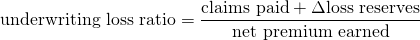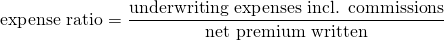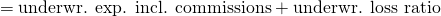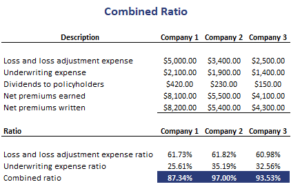# Combined ratio

The combined ratio (CR) in insurance is an important measure that is used to assess the profitability of Property & Casualty (P&C) Insurance companies. The combined ratio is the sum of the underwriting loss ratio and the expense ratio. It can be used to determine whether the current market is hard or soft.

On this page, we discuss the underwriting loss ratio and the expense ratio. Together they make up the CR. We also discuss the interpretation of the CR and how it relates to the pricing environment in P&C insurance.

## Combined ratio insurance definition

How to calculate the combined ratio? As we mentioned in the introduction, the ratio consists of two parts. So, let’s have a look at the formulas for both components.

The underwriting loss ratio is defined as followsThe second component is the underwriting expense ratioThe sum of both ratios is the combined ratioNote that the denominator is different in the ratios that make up the CR.

## Insurance combined ratio interpretation

How to interpret the ratio? If the ratio is higher than a 100%, then it means that the insurance company has an underwriting loss. In that case, margins are slim or negative and the market is experiencing a soft pricing period.  In the opposite case, we say the insurance market is experiencing a hard pricing period.

Both components of the CR can also be interpreted separately. The underwriting loss ratio measures the relative efficiency of the company’s underwriting standards. The underwriting expense ratio measures the efficiency of the company’s operations.

## Combined ratio insurance example

Finally, let’s go over the calculation by applying the formulas to a numerical example. The following table calculate the combined ratio for a P&C company. The data needed to calculate the three ratios are typically reported in the annual reports of  insurance companies. The Excel spreadsheet used can be downloaded a the bottom of the page## Summary

We discussed the combined operating ratio calculation using a simple numerical example. This metric is commonly used to value P&C insurance companies and can easily be calculated using an Excel spreadsheet.